# Math Practice Topic: Compare Rectangle Area and Perimeter

 Description: Calculate the rectangle area and perimeter. Express them in correct units. Adaptive Learning Progression: The side lengths get bigger. Start using MathScore for free

## Sample Levels (out of 4)

Find the area. Answer with proper units.

 1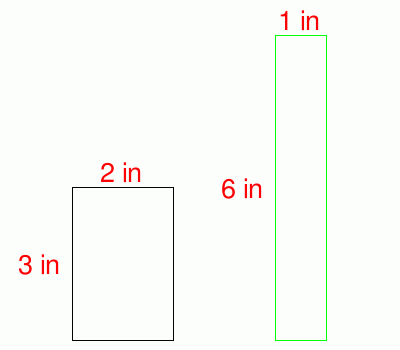Area of either Rectangle = Perimeter of Black Rectangle = Perimeter of Green Rectangle =

Find the area. Answer with proper units.

 1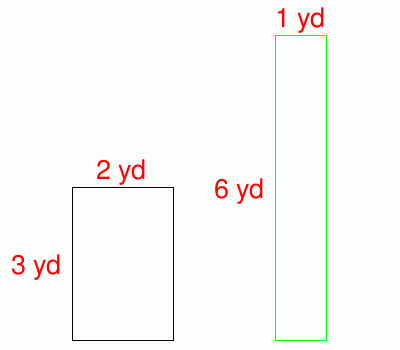Area of either Rectangle = Perimeter of Black Rectangle = Perimeter of Green Rectangle = 2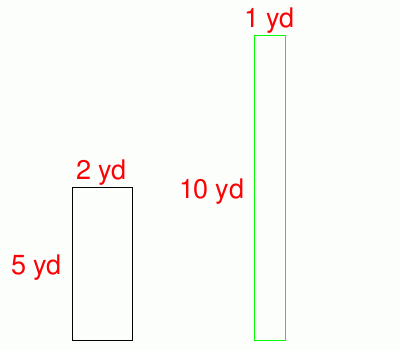Area of either Rectangle = Perimeter of Black Rectangle = Perimeter of Green Rectangle = 3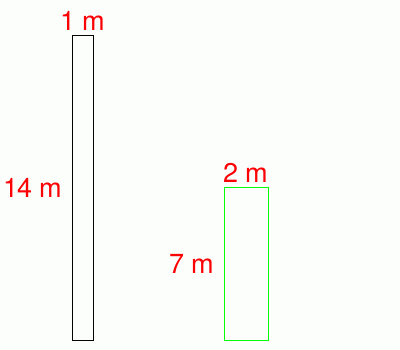Area of either Rectangle = Perimeter of Black Rectangle = Perimeter of Green Rectangle = 4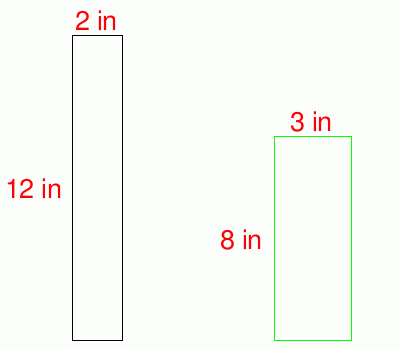Area of either Rectangle = Perimeter of Black Rectangle = Perimeter of Green Rectangle = 5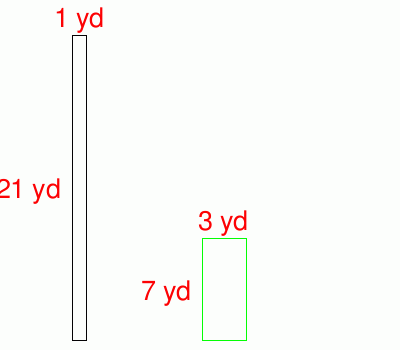Area of either Rectangle = Perimeter of Black Rectangle = Perimeter of Green Rectangle =